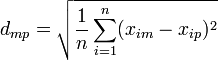Multidimensional Scaling (MDS)

Multidimensional Scaling (MDS) can be conducted in Q by:

Required data

Multidimensional scaling requires a Number - Multi question to be selected in the blue drop-down menu and RAW DATA in the brown drop-down menu. If data is not a distance matrix, Q automatically converts it into a distance matrix (this is discussed in more detail below).

Interpretation

The closer two variables are on a map, the more similar their data. Figure 90 shows an MDS chart based on six attitudinal variables, each measuring how much people like six brands of cola (Hate to Love). We can see from the chart that Coca-Cola is relatively isolated, whereas Diet Coke and Coke Zero have, relatively, similar ratings (i.e., people that like one are more likely to like another) and, similarly, the Pepsi brands cluster together, with Pepsi closest to Coca-Cola and the diet Pepsis closes to the diet Coke brands.

Technical details

Classic (metric) multidimensional scaling of the distance matrix (see Torgerson, W. S. (1958). Theory and Methods of Scaling. New York: Wiley).

Distances are computed between the mth and pth variable using:$d_{mp}=\sqrt{\frac{1}{n} \sum^n_{i=1} (x_{im}-x_{ip})^2 }$